## Derive the equation of motion of the block of mass m1 in terms of its displacement x. The friction between the block and the surface is negl

Question

Derive the equation of motion of the block of mass m1 in terms of its displacement x. The friction between the block and the surface is negligible. The pulley has negligible inertia and negligible friction. The cylinder has a mass m2 and rolls without slipping. Hint: First determine the equivalent mass me of the system.

in progress 0
5 months 2021-08-27T22:39:51+00:00 1 Answers 83 views 0

the equivalent mass :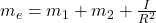the equation of the motion of the block of mass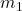in terms of its displacement is =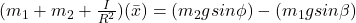Explanation:

Let use m₁ to represent the mass of the block and m₂ to represent the mass of the cylinder

The radius of the cylinder  be = R

The distance between the center of the pulley to center of the block to be = x

Also, the angles of inclinations of the cylinder and the block with respect to the ground to be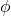and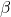respectively.

The velocity of the block to be = v

The equivalent mass of the system =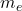In the terms of the equivalent mass, the kinetic energy of the system can be written as: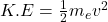————— equation (1)

The angular velocity of the cylinder =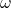:  &

The inertia of the cylinder about its center to be = I

The angular velocity of the cylinder can be written as: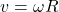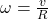The kinetic energy of the system in terms of individual mass can be written as: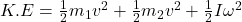By replacingwith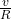; we have: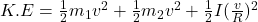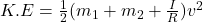—————— equation (2)

Equating both equation (1) and (2); we have:Therefore, the equivalent mass :which is read as;

The equivalent mass is equal to the mass of the block plus the mass of the cylinder plus the inertia by  the square of the radius.

The expression for the force acting on equivalent mass due to the block is as follows: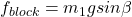Also; The expression for the force acting on equivalent mass due to the cylinder is as follows: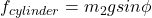Equating the above both equations; we have the equation of motion of the  equivalent system to be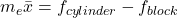which can be written as follows from the previous derivationsFinally; the equation of the motion of the block of massin terms of its displacement is =